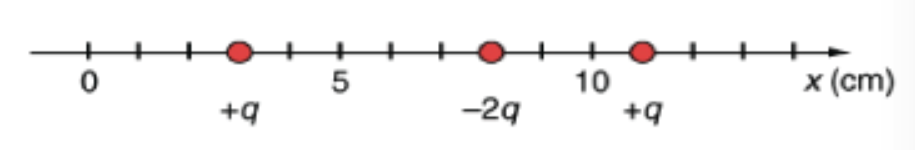# Problem: As shown in the figure, there are 3 point charges along the x-axis located at 3, 8, and 11 cm.What is the x-component of the force on the charge located at x = 8 cm given that q = 0.85 uC in N? Fx = ?

###### FREE Expert Solution

Force:

$\overline{){\mathbf{F}}{\mathbf{=}}\frac{\mathbf{k}\mathbf{q}\mathbf{Q}}{{\mathbf{r}}^{\mathbf{2}}}}$

Force due to the charge at 3 cm is acting in the negative x-direction.

r = 8 - 3 = 5 cm.

F3 = (9 × 109) (q)(-2q)/(5 × 10-2)2 = - (9 × 109) (2)(0.85  × 10-6)2/(5 × 10-2)2 = - 5.20 N

90% (498 ratings)###### Problem Details

As shown in the figure, there are 3 point charges along the x-axis located at 3, 8, and 11 cm.What is the x-component of the force on the charge located at x = 8 cm given that q = 0.85 uC in N?

Fx = ?